Páginas

Coupling between cw lasers and a frequency comb in dense atomic samples - Theory

1. Introduction
2. Experiment
3. Theory

In order to understand the fundamental aspects behind the experimental results of section 2, we consider the simplest possible theory. We model the atom as a four-level system, with two ground states and two excited states (see figure 5). The two ground states, correspond to the two hyperfine levels of the 5S1/2 manifold of rubidium. They are essential in the theory in order to allow for the various optical pumping conditions of the system as the experimental parameters are changed. The two excited states, represent different levels of the 5P3/2 manifold of rubidium, and are introduced to account for the orthogonal polarizations of the diode and femtosecond lasers. In this way, both lasers may interact with atoms in the same ground state, but, as occurs in the experiment, they do not connect the same transition.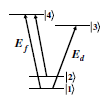These electric fields can be written as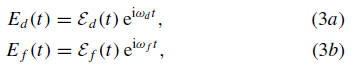For the train of femtosecond pulses, theenvelope can be written as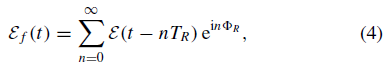We do not consider the phase fluctuations of the diode and fs lasers in our treatment. These fluctuations occur on a time scale of the order of, or larger than, the time required for the atomic ensemble to reach its stationary state, as will be shown below. The phase fluctuation time scales are given by the inverse of the respective linewidth of a single mode of the lasers, about 1 µs for the diode laser and much larger than that for the fs laser (see footnote 2). During the time evolution to the ensemble’s stationary state, the two lasers have a roughly well-defined relative phase, which we consider here, for simplicity, to be zero.

The temporal evolution of the atomic state is given by the set of Bloch equations for the system. In the rotating wave approximation and defining the Rabi frequencies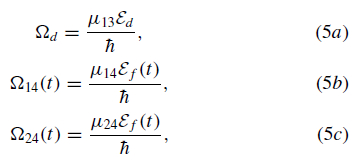the Bloch equations can be written as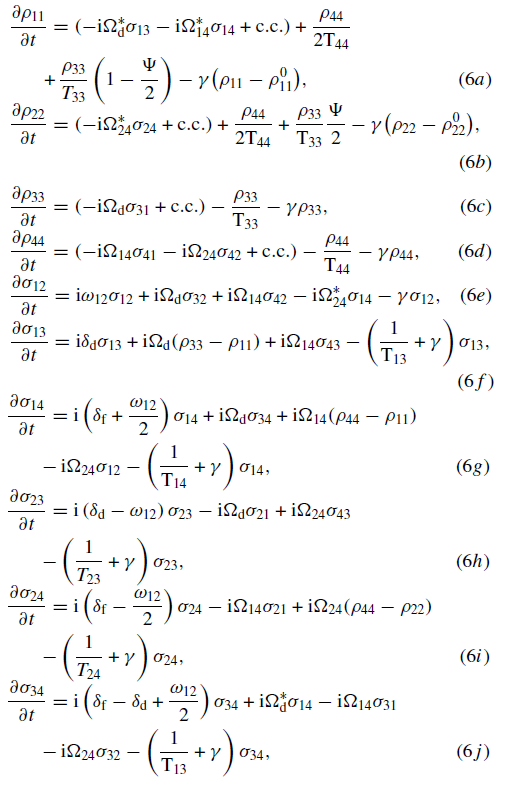The finite interaction time due to the escape of atoms from the interaction region is accounted for by the relaxation rate. This loss of atoms is compensated by the arrival of new atoms in the ground state at the same rate. The transition is open if the state 3 can decay into either states 1 and 2, and is closed if it only decays into state 1. As we will see, in the case of an open transition, competition between the two lasers is present, depending on their relative intensities, while for a closed transition, the optical pumping process is driven only by the fs laser.

The following parameters were used in the numerical calculations: the central frequency of the femtosecond laser was fixed at 780 nm, with pulses of temporal width 150 fs, and a repetition period of 13 ns. The lifetimes of the levels 3 and 4, corresponding to two levels of the 5P3/2 manifold, are taken as 26.2 ns. The energy separation between the two ground states 1 and 2 is 6835 MHz . For the homogeneous broadening, we consider only radiative processes and take 52.4 ns. For a beam waist of ~ 100 µm, and at room temperature, we consider an interaction time of 400 ns.
The time evolution of the atomic populations and coherences is obtained by solving the set of coupled differential equations, equations (6), for all orders of the fields. The Bloch equations were integrated using a standard fourth-order Runge–Kutta method with adaptive step size . For the numerical calculations, we consider a train of pulses interacting with an atom initially in the ground state, with µ13 = µ14 = µ24.

We start by considering the weak-field limit for both fs and cw lasers and investigate the effect of increasing the fs laser intensity on the diode-laser transmission. In sections 3.1 and 3.2 we investigate the variation of the cw-laser power and the dependence on the atomic density, respectively.
To compare with our experimental results we first note that the probe-beam transmission variation is related to the absorption of the diode beam by the medium. The average power lost by the probe beam per unit volume is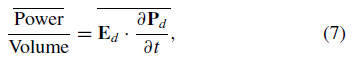For an ensemble of atoms, the absorption is therefore given by the imaginary part of the polarization, which is proportional to the coherence between the level 1 and 3, excited by the diode laser.

The calculations are performed for different atomic velocity groups (different detunings d). In order to compare the intensity of the two beams we define the quantity which is a parameter that indicates how strongly the transition is driven during one repetition period of the laser.

For each frequency of the cw laser, we take into account only the contribution of the atoms that are on resonance with the diode laser, and weight the curves by the Doppler profile. For low femtosecond-laser intensities (figure 6(a)), we clearly observe a velocity selective population transfer between the two lower states, induced by the frequency-comb excitation. As the diode laser only interacts with the ground state 1, we obtain gain or loss depending if the fs laser decreases or increases the population of this state. It means that, for atomic velocity groups for which a tooth of the fs-laser frequency comb is resonant with the 1 -4 transition, the population of level 1 decreases. On the other hand, for atomic velocity groups for which the fs frequency comb is resonant with the 2 -4 transition, population is transferred from level 2 to level 1. When the fslaser intensity increases (figure 6(b)) we continue observing the frequency comb impressed in the Doppler profile, but now, the optical pumping between the two lower states is lost due to power broadening induced by the femtosecond laser .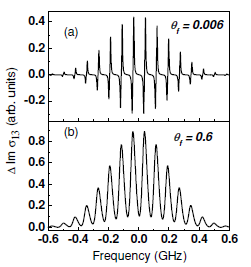3.1. Variation of the cw-laser power

We also investigate the diode-laser transmission variation as its intensity is increased. In the case of a weak fs beam, the calculated fractional population variation is shown in figure 7, for the two ground states, 1 (figures 7(a) and (c)) and 2 (figures 7(b) and (d)), when the pulse area of the cw beam is smaller or greater than the pulse area of the fs beam. In this figure the fs-laser intensity is fixed and the diode electric-field amplitudes are Ed = 4 V/m (figures 7(a) and (b)) and Ed = 5 Vm-1 (figures 7(c) and (d)). We see that the population of state 1 can increase or decrease depending on the relative pulse areas between the two beams, indicating that the strongest beam dominates the optical pumping process. To observe this optical pumping competition between the two beams, we consider a velocity group for which the atoms are resonant simultaneously with thetwo beams, and perform the calculations for the open diodelaser transition and with the fs frequency-comb resonant with the 2 - 4 transition.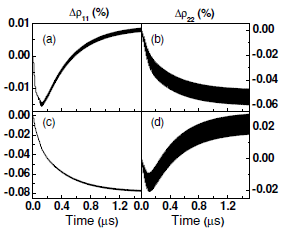Figure 8 shows the population of the excited state 4 as a function of the atomic velocity distribution for high intensity and fixed frequency of the cw laser. The population distribution. The cw diode-laser frequency is fixed. As described previously, the periodic structure with two peaks observed for low-femtosecond-laser intensity (as in figure 6(a)), is a consequence of the two resonant transitions, 1 - 4 and 2 - 4, with different modes of the fs pulse train. For a diode-laser intensity that corresponds to an electric field amplitude of the order of Ed = 1 kV/m, we observe that the various atomic velocity groups are still sensitive to the fs-laser frequency comb. However, the Stark shift of the 1 - 3 atomic transition by the diode laser displaces the printing of the comb teeth in the Doppler profile which are resonant with the 1 - 4 transition, around the cw-laser frequency, as shown in the region around the dashed lines in figure 8. We also note that the comb teeth resonant with the 2 - 4 transition are not displaced, as these two levels are not directly connected with the diode laser. Following the dashed lines in figure 8 it is clearly seen that the indicated atomic velocity groups will be resonant or not with the fs-laser frequency comb depending on the cw diode intensity. This means that the accumulative effect observed on the atoms with these specific velocities will be destroyed due to the Stark shift of the atomic transition. On the other hand, as shown in figure 8, there will be other atomic velocity groups that will change from destructive to constructive interference condition, depending on the displacement due to the Stark effect.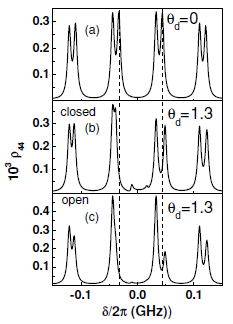The transmission variation of the diode beam induced by the fs laser was calculated for the fixed fs pulse area 0.6, varying the cw-laser pulse area by one order of magnitude: from 0.05 (Ed = 40 V/m) ((a) and (b)) to 0.5 (Ed = 400 V/m) ((c) and (d)). First, it is important to note that the curve in figure 9(a) was obtained for the same parameters as used in figure 6(b), except for the diode intensity, that is now three orders of magnitude greater.

To take into account effects such as power broadening and Stark shift, due to high cw-laser intensity, the curves in figure 9 were obtained integrating, for each position of the diode-laser frequency, the contribution of all atomic velocity groups within the linewidth of the 1 - 3 transition. For very low cw laser intensity, the integration is over the natural linewidth of the transition. However, for the intensities used in figure 9, the integration regionmust be of the order of the power-broadened linewidth. Comparing figure 6(b) with figure 9(a) we clearly see the blurring of the frequency comb printed in the Doppler profile as a result of the extra power broadening of the atomic transition by the cw laser.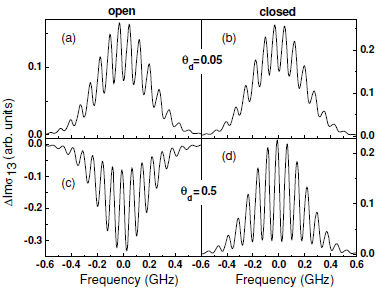For low cw-laser intensities, we obtain amplification of the diode beam induced by the fs beam for both kinds of transitions. As the cw-laser intensity increases, we find an intensity region where the two kinds of transitions, closed and open, present different behaviour with respect to absorption and amplification. While for the closed transition we continue to obtain diode amplification, for the open transition the fs beam induces an increase of the absorption of the diode beam for all frequencies within the Doppler profile. This distinct behaviour can be understood if we note that for the closed transition only the fs beam can transfer population between the states 1 and 2. However, for the open transition, there is a competition between the two lasers in the population transfer process (as shown in figure 7) and the diode laser may prevail when its area is large enough.
To simulate our experimental results, we take into account this distinct behaviour depending on the nature of the atomic transition. We also consider the frequency separation and the relative transition dipole moments of the hyperfine transitions. In figure 10 we present the simulations for a fs electric-field amplitude of Ef = 40 MV/m and three values of the diode electric field amplitude: (a) Ed = 150 V/m, (b) Ed = 1000 V/m and (c) Ed = 2000 V/m. These values correspond to the experimental conditions of the results shown in figure 2, at fixed temperature, with an average power of 350 mW for the fs laser and approximately the same ratio between the diode electric-field amplitudes. The curves were obtained by integrating the contribution of all atomic velocity groups within the power-broadened linewidth of the 1 - 3 transition, according to each value of the diode electric-field amplitude. Also, for each atomic velocity group, we took the average value, over a period, of the coherences, after the system reaches a stationary state. For low atomic densities as in figure 2, the linear absorption is negligible. The visibility of the modulations decreases when the diode electric-field amplitude increases, while the absorption of the diode beam induced by the fs laser increases throughout the whole Doppler profile, in good agreement with the experimental results.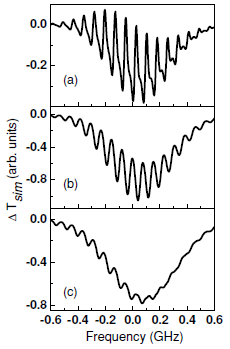3.2. Dependence on the atomic density

The experimental results observed for different atomic densities can be understood qualitatively if we take into account the distinct effects of the open and closed transitions on the changes introduced in the cw beam intensity. First, we note in figures 3(e)–(h) a decrease of the diode beam intensity due to absorption by the rubidium vapour as the atomic density increases. For high cw beam intensity and low atomic densities, however, this decrease is negligible (see figure 3(e)) and the cw beam transmission is also practically frequency independent. Under these conditions, from our theoretical results of section 3.1 (see figure 10), we expect that the open and closed transitions should display the same behaviour, i.e. an increase of the probe beam absorption induced by the fs beam. This is in agreement with the results for the cw-laser frequency scanned over the Fg = 1 - Fe = 0, 1, 2 hyperfine transitions, as shown in figure 3(a); in fact, negative values for the transmission changes of the cw beam are observed for all frequencies in the Doppler profile.
As the atomic density increases, the cw laser is more absorbed and its intensity decreases in the interaction region, the centre of the cell. Positive values for transmission are then observed on the red side of the Doppler profile (see figures 3(b)–(d)). As predicted in figure 9, this situation corresponds to an increase of the cw beam transmission induced by the fs beam in the closed transition, Fg = 1 to Fe = 0, while negative values for transmission continue to be observed in the frequency region of the open transitions, Fg = 1 - Fe = 1 and 2. The influence of the nature of the transition driven by the diode laser is reinforced by the results displayed in figure 4(b) for the cw laser frequency scanned over the Fg = 2 Fe = 1, 2, 3 hyperfine transitions. In this figure, we clearly see that positivevalues for the probe beam transmission variation appear now on the blue side of the Doppler profile, corresponding again to the frequency region where the closed transition dominates. These features indicate that the distortions observed in the probe-beam transmission variation due to the fs laser and as the atomic density increases (figures 3 and 4) are well explained by the distinct behaviour of these two kinds of transitions driven by the diode laser.